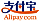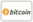Portada del libro de Series Approximation in the Applied Sciences Problems
Título del libro:

# Series Approximation in the Applied Sciences Problems

Scholars' Press (2014-11-25 )apto para el cupón
ISBN-13:

### 978-3-639-70985-8

ISBN-10:
3639709853
EAN:
9783639709858
Idioma del libro:
Inglés
Notas y citas / Texto breve:
The aim of this book is to present new series approximation methods for solving linear and nonlinear differential equations which arising in applied Sciences. These procedures applied on fluid dynamics problems, population model equations, oscillator problems, fractional order equation, batch reactor equation and the Riccati's differential equations. It is interesting to note that these methods give the analytic and numerical results.
Editorial:
Scholars' Press
Sitio web:
http://www.scholars-press.com
Por (autor):
Najeeb Alam Khan
Número de páginas:
196
2014-11-25
Stock:
Disponible
Categoría:
Matemáticas
Precio:
79.90 €
Palabras clave:
fractional calculus, Nonlinear Sciences, series solution### Enlaces